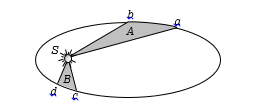A satellite which is geostationary in a particular orbit is taken to another orbit. Its distance from the centre of earth in new orbit is 2 times that of the earlier orbit. The time period in the second orbit is

(a) 4.8 hours                         (b) $48\sqrt{2}$ hours

(c) 24 hours                           (d) $24\sqrt{2}$ hours

Concept Questions :-

Satellite
High Yielding Test Series + Question Bank - NEET 2020

Difficulty Level:

The ratio of the K.E. required to be given to the satellite to escape earth's gravitational field to the K.E. required to be given so that the satellite moves in a circular orbit just above earth atmosphere is

(a) One                           (b) Two

(c) Half                            (d) Infinity

Concept Questions :-

Escape velocity
High Yielding Test Series + Question Bank - NEET 2020

Difficulty Level:

An astronaut orbiting the earth in a circular orbit 120 km above the surface of earth, gently drops a spoon out of space-ship. The spoon will

(a) Fall vertically down to the earth

(b) Move towards the moon

(c) Will move along with space-ship

(d) Will move in an irregular way then fall down

Concept Questions :-

Satellite
High Yielding Test Series + Question Bank - NEET 2020

Difficulty Level:

The period of a satellite in a circular orbit around a planet is independent of

(a) The mass of the planet

(b) The orbital radius of satellite  around the planet

(c) The mass of the satellite

(d) All the three parameters (a), (b) and (c)

Concept Questions :-

Satellite

Difficulty Level:

Two identical satellites A and B go round a planet P in circular orbits having radii 4R and R respectively. If the speed of the satellite A is 3V, the speed of the satellite B will be ?

(a) 12 V                     (b) 6 V

(c) $\frac{4}{3}v$                       (d) $\frac{3}{2}v$

Concept Questions :-

Orbital velocity
High Yielding Test Series + Question Bank - NEET 2020

Difficulty Level:

A geostationary satellite

(a) Revolves about the polar axis

(b) Has a time period less than that of the near-earth satellite

(c) Moves faster than a near-earth satellite

(d) Is stationary in the space

Concept Questions :-

Satellite

Difficulty Level:

A small satellite is revolving near earth's surface. Its orbital velocity will be nearly

(a) 8 km/sec                       (b) 11.2 km/sec

(c) 4 km/sec                       (d) 6 km/sec

Concept Questions :-

Orbital velocity

Difficulty Level:

The distance of neptune and saturn from sun are nearly ${10}^{13}$ and ${10}^{12}$ meters respectively. Assuming that they move in circular orbits, their periodic times will be in the ratio

(a) $\sqrt{10}$                                                (b) 100

(c) $10\sqrt{10}$                                             (d) $1/\sqrt{10}$

Concept Questions :-

Kepler laws
High Yielding Test Series + Question Bank - NEET 2020

Difficulty Level:

The orbital velocity of an artificial satellite in a circular orbit just above the earth's surface is v. For a satellite orbiting at an altitude of half of the earth's radius, the orbital velocity is

(a) $\frac{3}{2}v$                      (b) $\sqrt{\frac{3}{2}}v$

(c) $\sqrt{\frac{2}{3}}v$                    (d) $\frac{2}{3}v$

Concept Questions :-

Orbital velocity
High Yielding Test Series + Question Bank - NEET 2020

Difficulty Level:

The figure shows the motion of a planet around the sun in an elliptical orbit with sun at the focus. The shaded areas A and B are also shown in the figure which can be assumed to be equal. If ${t}_{1}$ and ${t}_{2}$ represent the time for the planet to move from a to b and d to c respectively, then

(a) ${t}_{1}<{t}_{2}$

(b) ${t}_{1}>{t}_{2}$(c) ${t}_{1}={t}_{2}$

(d) ${t}_{1}\le {t}_{2}$

Concept Questions :-

Kepler laws